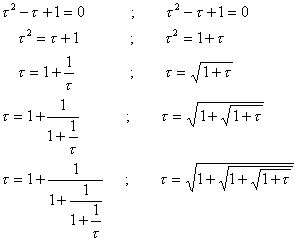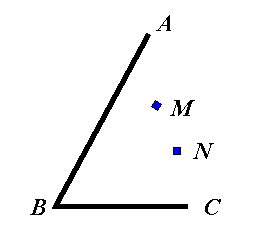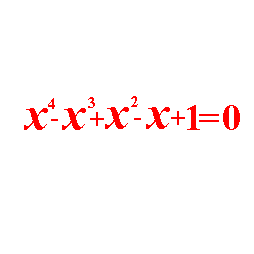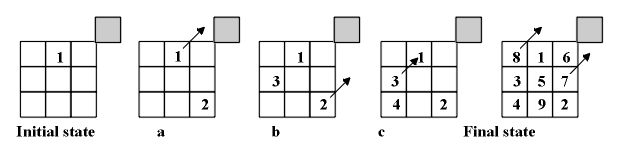On Symmetry in School Mathematics

Tommy Dreyfus1)
&
Theodore Eisenberg2)

Center for Technological Education,1)
Holon, Israel
tommy.dreyfus@weizmann.ac.il

Ben-Gurion University,2)
Beer-Sheva, Israel
eisen@black.bgu.ac.il

The Ubiquity of Symmetry

Everywhere we turn we can see symmetrical relationships. They are both visual and audio, and they are so pervasive in our daily lives that one is led naturally to wonder if the notion of symmetry is innate in human beings. It is almost as though the notion of symmetry is built into us as a standard against which we measure aesthetic appeal to assess both mental and physical constructs. Hargittai and Hargittai in their text Symmetry: A Unifying Concept illustrate how deeply seated and ubiquitous symmetrical relationships are through hundreds of photographs of man-made objects, from examples in architectural symmetry, to those found in nature, as exemplified by the markings on the wings of a butterfly. The notion of symmetry is itself a mathematician's dream, for point and line symmetries which have been extensively studied in their own right, have been generalized and applied to almost every area of mathematics, even school mathematics. Moreover, entire domains of mathematics, such as group theory have arisen out of the study of symmetry.

Algebra, geometry, trigonometry and calculus are four main domains of school and collegiate mathematics. And in each of these domains, students are introduced to generalizations on the notions of symmetry; e.g., in algebra they are introduced to symmetric functions, symmetric determinants, symmetric groups, symmetric systems of equations and to symmetric forms, as in the symmetric form of the equatation of a line; in geometry they meet the notions of point and line symmetry, and n-fold symmetry. In trigonometry the students meet basic symmetric relations like cosa = sin(90o-a), and at a more advanced level, the notions of symmetric spherical triangles. In elementary calculus they again see that symmetry plays a pivotal role in applying integration techniques and in working with differential forms. The centrality of symmetry as a notion in and of itself, not to mention its use as a heuristic in problem solving, is easily documented in general mathematics, and in school mathematics too. But whether or not there is a natural, innate, gravitation towards symmetry is an open question, although many giants in mathematics and the physical sciences (Poincare, 1913;   Einstein, 1935Weyl,  1952; Polya, 1962 and Penrose, 1974) have addressed their own propensities for symmetry and aesthetics, individually saying that they believe it to be one of the driving forces behind their work.

Symmetry as an Aesthetic Metric

It is well known that there seems to be a small set of real numbers which appeal to our psyche more than other numbers. E.g., more than a hundred years ago the psychologist Gustav Fechner made literally thousands of measurements of rectangles commonly seen in everyday life; playing cards, window frames, writing papers, book covers, etc., and he noticed that the ratio of the length to the width seemed to approach the golden ratio t=(1+Ö5)/2. He then presented rectangles to hundreds of individuals and asked them to choose one, with no selection criteria specified. He claims that they disproportionately chose rectangles, the ratio of whose sides was close to the golden ratio. He then questioned the innateness of this number in the psyche of man; a number which was already known to be ubiquitous in nature, science and mathematics. According to Huntley (1970), Fechner's experiments were repeated by Witman, by Lalo and again by Thorndike, each a leading psychologist of his time, and each obtained similar results. Huntley's listing of Fechner's data is presented in Figure 1.Figure 1

The golden ratio is a number closely tied to symmetry. The ratio is obtained as follows. Given the line segment AB there are two special points C and D on it that divide the line segment into the golden ratio. One of these points C is an interior point to the segment AB. The other point D is an exterior point to the segment; i.e., the segment AB must be extended to reach it.Figure 2

The Golden Ratio t, is defined as follows:

 t = AC CB = AB AC ; AD BD = BD AB = t
 t = AC CB = AC+CB AC ; AB+BD BD = BD AB = t
 t = AC CB = 1 + CB AC ; AB BD +  1 = BD AB = t
 t = 1 + 1 t ; 1 t + 1 = t
 t2 - t -1 = 0   ;   0 = -1 -t + t2
 t = 1 + Ö5 2 .

Constructing the points C and D for given line segment AB is an instructive exercise which is closely associated with the Appolonian circle for fixed points A and B; the Appolonian circle is the locus of a point P such that AP/PB is a constant k>0, ką 1. How do we find points C and D? With B as the center, draw a circle of arbitrary radius r; now with A as the center draw a circle of radius tr. If these two circles do not intersect, choose another value for the radius r, and repeat the procedure. Let the circles intersect at P. Note that AP/PB=t. Bisect ĐAPB internally and externally. Let the internal bisector meet line segment AB at C; and let the external bisector meet AB extended at D. We have now found the two desired points for the golden ratio. If the length of AB is one, then AC=1/t, CB=1/t2 and BD=t.

The aesthetic appeal of the golden ratio and its ties to the Fibonacci sequence, as well as its far reaching connections to nature and science are well documented in the literature (Huntley, 1970; Herz-Fischler, 1998; Dunlap, 1998). But the connection of this number to the human psyche, in the spirit of Fechner's work with investigating our attractions toward it, is an open question. Nevertheless, there are many aspects of symmetry which are embedded in the golden ratio and which are instructive for students to study. (Space limitation allow us to only mention a few.)

The two points C and D are said to divide the line segment AB into its mean and extreme ratio - and there is no other set of points which does this. I.e., there is no other set of points X and Y on segment AB such that

 AX XB = AB AX = t = AY YB = YB AB .

Perhaps the ratio is not connected to our subconsciousness, but it and its reciprocal, ((Ö5 - 1)/2), are somehow connected to our aesthetic attraction towards simplicity and minimalism.

Hidden symmetries in the golden ratio abound. For example, t, the golden number, satisfies the equation t2 -t-1 = 0.We now have the simplest continued fraction and root expansion known to man; each of which can be continued on ad infinitum in a systematic, patterned way. And as soon as one thinks of patterns, one thinks of symmetry. So here we have a systematic (symmetric) way to represent an irrational number, that in and of itself is almost a contradiction of terms, for irrational numbers do not have systematic decimal representations; yet with the notions of continued fractions and infinite square roots there is a certain symmetry to them.

Whether or not we have a subconscious gravitation towards symmetry, and special numbers such as the golden ratio, is admittedly a hazy area and perhaps best left to psychologists to investigate. But like learning to appreciate art and music, where one learns what to look for with respect to a painting, and what to listen for with respect to a piece of music of a particular period, one must be taught how to look for symmetrical relationships; gravitation towards symmetry might happen naturally, but learning how to utilize symmetry must be taught (Dreyfus and Eisenberg, 1990).

Teaching to Utilize Symmetry

At the most basic level it helps to look at texts like the one written by the Hargittais, where the ubiquity of point and line symmetries are vividly pointed out and awake our sensitivity to geometrical symmetry. But the notion of symmetry enters many domains of school mathematics other than geometry. One of these domains is problem solving, where symmetry must be seen or imposed on a problem to effect its solution. Another domain is in concept formation, where it is often advantageous to think of basic mathematical notions in terms of symmetrical properties which surround them.

Figure 3 lists three problems whose solutions depend on symmetry. Do you see how to solve them and to generalize the problem? It has been our experience that most students cannot solve these problems, because they do not use symmetry as a heuristic tool. (Partial answers are presented at the end of this paper.)

(a)(b)(c)Figure 3: a) Find a point P on AB and point Q on BC so that MP + PQ + QN is minimal; b) Solve the equation; c) Given a billiard table 4×3. A ball stands in the lower left-hand corner and travels at the angle of 45°. This ball will end up in the upper left-hand pocket. Generalize.

But symmetry need not be limited to geometry and algebra. Let us consider the notion of Magic Squares.

Magic Squares: The idea underlying magic squares is to put n2 integers into the cells of an n×n square so that the sums obtained by adding the numbers in each column, each row and each diagonal are equal. When we restrict the numbers to the positive integers 1,2,3,...,n2 we call the magic square a normal magic square of order n.

There are many problems associated with magic squares. E.g.,

• Show that it is impossible to have a magic square of order two.
• Show the center cell in a 3×3 normal magic square must be 5.
• Showthat it is impossible to have the number 1 in a corner cell of a normal 3×3 magic square.
• What must be the sum of each row in a 10×10 normal magic square?

The following algorithm can be extended to all magic squares of odd order; we exemplify it in Figure 4 for the 3×3 case. Start with 1 in the top middle cell. Now move along the diagonal, always moving upward and to the right. If in so moving, we find ourselves outside of the square's frame at the top, we continue filling in the cells of the square at the bottom of that column (Fig 4a). If we find ourselves outside the frame on the right, we move to the first cell on the left of that row (Fig.4b). If a cell is blocked within the frame itself because we have already filled it in, we drop to the cell immediately under the one from which we came, fill it in (Fig.4c) and continue filling in the square moving upward and to the right.Figure 4

There are several ways to see that the square is really magic. One way is to actually check the sums in each row, column and diagonal. Another is a little easier; e.g., to check to see if a 5×5 square is indeed magic, write each number in each cell in base 5. Now from the number in each cell subtract one from its base 5 representation. What remains is all combinations of two digit numbers in base five. It can be seen that each row and column and one of the diagonals are some permutation of the same digits. In other words, one need not do the actual computations, but simply check to make sure that all digits are there.

Students love to play with magic squares, but they are often unable to construct ones which are not normal. Here is where symmetry can enter because all magic squares of order 3 are of a particular form as shown in Figure 5.Figure 5

Students are often amazed at how simple this is for them and they often try to generalize the method of building symmetry along the diagonals of higher ordered squares. This exercise opens up many doors for discussion, building in symmetry to effect a solution and degrees of freedom are just two avenues for deeper work.

Symmetry in cubic polynomials: There are two ways to prove that every cubic polynomial is symmetric about its point of inflection. A cubic polynomial is one of the form f(x) = ax3 + bx2 + cx + d and its general graph is listed in Figure 6.Figure 6

Its point of inflection is at

(-b/3a, f(-b/3a)),

So, using its graph to guide us we wish to show that:

f((-b/3a)-x)-f(-b/3a) = f(-b/3a)-f((-b/3a)+x)

The algebra is not easy but straightforward.

Another way to do the problem is to play on its symmetry from the start. First we look at the graphs of y = x3 and y = (x-1)x(x+1). Each of these graphs has its point of inflection on the y-axis, and the students easily prove that the graphs are symmetric with respect to their point of inflection. We then talk about the effect of adding a constant to the above equations, y = x3+k, y = (x-1)x(x+1) + k. The students immediately realize that the effect of adding a constant to equations of this type simply pushes the graph up or down the y-axis; their points of inflection remain on the y-axis and thus the symmetry remains intact. The students thus realize that an equation of the form y = x3 + Bx + C has a point of infection on the y-axis and that its graph is symmetric about it.

We then return to the given equation and ask if there is some way to transform it into one that will result in a cubic of the form y = z3 + Bz + C. The transformation x = z - b/(3a) will move the graph horizontally so that its point of inflection is on the y-axis. In effect this gives another proof that cubic equations are symmetric about their point of inflection.

Summary

In this paper we have tried to show that symmetry is a concept which can be exploited and used as a red thread connecting different branches and skills in school mathematics. But the main message we have tried to argue is that symmetry must be taught. It is too useful and important of a topic to let it develop casually, if at all, as one passes through the school curriculum.

Acknowledgment

We would like to thank Slavik Jablan for the wonderful animation of the static diagrams we submitted to him, and for the suggestions he made for improving the original draft of this paper as well as for the encouragement he gave us along the way.

• Bibliography

Weyl, H. Symmetry. Princeton: Princeton University Press, 1952.

a) Let M* be the reflection of point M through AB. Similarly, let N* be the reflection of point N through BC. Let segment M*N* intersect AB and BC in points P and Q respectively. MP + PQ + QN is minimal. Do you see why?b) This is a symmetric polynomial. As such, we should focus on its middle term, namely x2. Since xą 0 we can divide the polynomial by x2.

 x4-x3+x2-x+1 = 0 Ţ x2-x+1-1/x+1/x2 = 0
Letting t = x+1/x and noting that t2-2 = x2+1/x2 transforms the equation into a quadratic which gives a surprising solution.

c) Systematically consider special cases. A conjecture for the general case will soon be apparent. An elegant way to prove the conjecture is to use symmetry, by repeatedly reflecting a table of given dimensions about its edges. The path of the ball becomes a straight line.